# Geometry

## 语法

` Geometry (geometry, inputs, {spatial_reference}, {has_z}, {has_m})`
 参数 说明 数据类型 geometry 几何类型：点、面、折线或多点。 String inputs 用于创建对象的坐标。数据类型可以是 Point 或 Array 对象。 Object spatial_reference 新几何的空间参考。(默认值为 None) SpatialReference has_z Z 状态：如果启用 Z，则为几何的 True，如果未启用，则为 False。(默认值为 False) Boolean has_m M 状态：如果启用 M，则为几何的 True，如果未启用，则为 False。(默认值为 False) Boolean

JSON
(只读)

String
WKB
(只读)

Bytearray
WKT
(只读)

String
area
(只读)

Double
centroid
(只读)

Point
extent
(可读写)

Extent
firstPoint
(只读)

Point
hasCurves
(只读)

Boolean
hullRectangle
(只读)

String
isMultipart
(只读)

Boolean
labelPoint
(只读)

Point
lastPoint
(只读)

Point
length
(只读)

Double
length3D
(只读)

Double
partCount
(只读)

Integer
pointCount
(只读)

Integer
spatialReference
(只读)

SpatialReference
trueCentroid
(只读)

Point
type
(只读)

String

## 方法概述

 方法 说明 angleAndDistanceTo (other, {method}) 返回到点或面的角度和距离的元组。 boundary () 构造几何边界。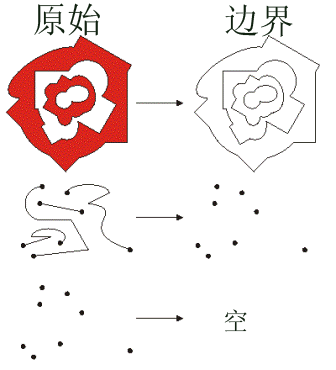buffer (distance) 在距几何的指定距离处构造一个面。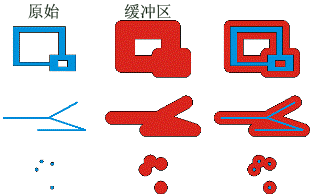clip (envelope) 构造几何体与指定范围的交集。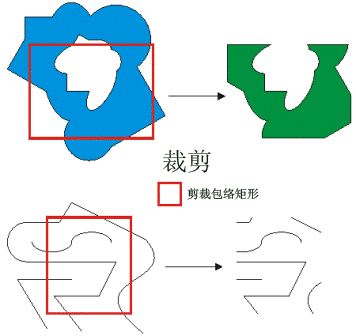contains (second_geometry, {relation}) 指明基础几何中是否包含比较几何。contains 与 within 相反。本图仅显示 True 关系。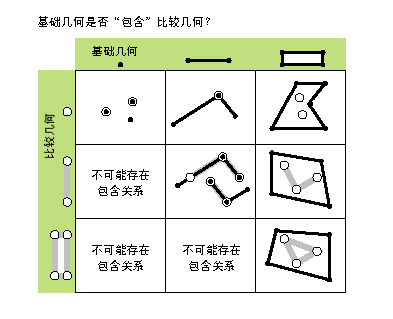convexHull () 构造具有最小边界多边形的几何，以便所有外角均为凸角。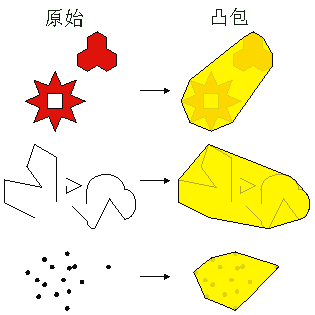crosses (second_geometry) 指明两个几何是否相交于较小形状类型的几何。 如果两条折线仅共用公共点（至少有一个点不是端点），则这两条折线交叉。如果折线和面在面（不等于整条折线）的内部共享一条折线或一个公共点（对于垂线），那么该折线与面交叉。本图仅显示 True 关系。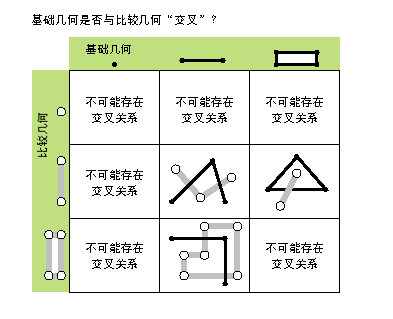cut (cutter) 将该几何分割到剪切折线的左右两侧。 剪切折线或面时，会从其与剪切折线的相交处将其分割。每一段被分类为剪切线的左侧或右侧。该分类基于剪切线的方向。目标折线中不与剪切折线相交的部分将作为该输入折线结果的右侧部分返回。如果未对几何进行剪切，则左侧几何将为空 (None)。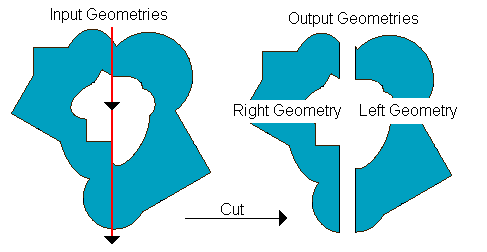densify (type, distance, {deviation}) 使用添加的折点 创建新的几何。 difference (other) 构造一个几何体，该几何体仅由基础几何所特有、而其他几何所没有的区域组成。下图显示当红色多边形为源几何时的结果。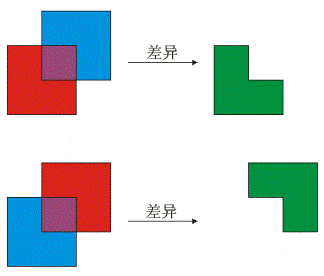disjoint (second_geometry) 指明基础几何和比较几何是否未共用任何点。如果 disjoint 返回 False，则两个几何相交。本图仅显示 True 关系。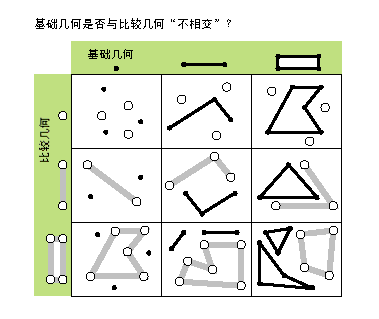distanceTo (other) 返回两个几何之间的最小距离。距离将采用几何空间参考的单位。如果两个几何相交，则最小距离为 0。两个几何必须具有相同的投影。 equals (second_geometry) 指示原几何和参照几何的 shape 类型是否相同并在平面中定义相同点集。这仅是 2D 的比较；已忽略 M 值和 Z 值。本图仅显示 True 关系。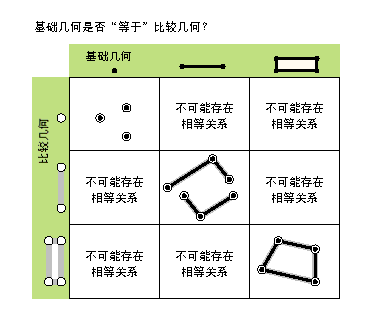generalize (max_offset) 使用指定的最大偏移容差来创建一个简化几何。 getArea ({type}, {units}) 使用测量类型返回要素的面积。 getLength ({measurement_type}, {units}) 使用测量类型返回要素的长度。 getPart ({index}) 如果指定了索引，则将返回几何特定部分的 Point 对象的 Array 对象。 如果未指定索引，则返回的 Array 对象将包含每个几何部分的 Point 对象的 Array。getPart 方法等同于建立对象索引；即 obj.getPart(0) 等同于 obj。 intersect (other, dimension) 构造作为两个输入几何交集的几何体。不同的维数可用于创建不同的 shape 类型。 对于同一 shape 类型的两个几何体，其交集为仅包含原始几何重叠区域的几何。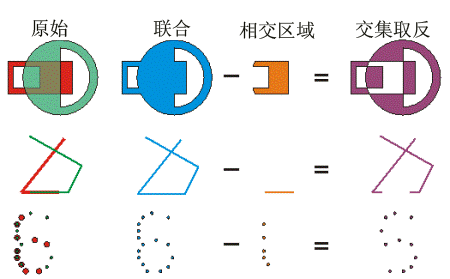为了更快地获取结果，请在调用 intersect 类之前先测试两个几何体是否 disjoint。 measureOnLine (in_point, {use_percentage}) 返回从此条线的起点到 in_point 之间的一个测量值。 overlaps (second_geometry) 指示两个几何的交集是否具有与其中一个输入几何相同的形状类型，并且不等于任一输入几何。 本图仅显示 True 关系。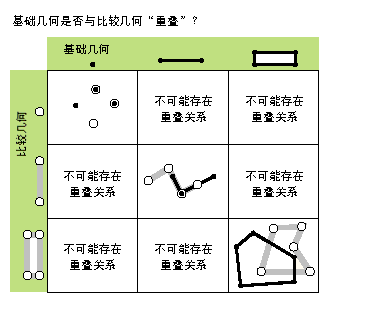pointFromAngleAndDistance (angle, distance, {method}) 使用指定的测量类型按给定的角度（以度为单位）和距离（采用几何空间参考的单位）返回点。 positionAlongLine (value, {use_percentage}) 返回线上距线起点指定距离处的点。 projectAs (spatial_reference, {transformation_name}) 定义几何投影，并应用相应的地理变换。对于工程，几何必须具有空间参考，并且没有未知的坐标系。 传递给该方法的新空间参考系统定义了输出坐标系。 如果两个空间参考均未知，则坐标将不会更改。 projectAs 方法不会更改 z 值和 m 值。 queryPointAndDistance (in_point, {as_percentage}) 在折线上找到离 in_point 最近的点，并确定这两点间的距离。同时返回关于 in_point 位于线的哪一侧以及最近点沿线的距离。 segmentAlongLine (start_measure, end_measure, {use_percentage}) 在起始测量值和结束测量值之间返回 Polyline。虽然与 Polyline.positionAlongLine 相似，但是会在折线的两点之间（而不是在单点）返回折线段。 snapToLine (in_point) 基于以该几何作为捕捉目标的 in_point 返回一个新点。 symmetricDifference (other) 构造一个几何体，该几何体由两个几何的并集减去其交集所形成。两个输入几何必须为同一 shape 类型。touches (second_geometry) 指示几何的边界是否相交。 当两个几何的交集不为空，但它们内部的交集为空时，说明两个几何接触。例如，仅当点与折线的一个终点重合时，才表示点与折线接触。本图仅显示 True 关系。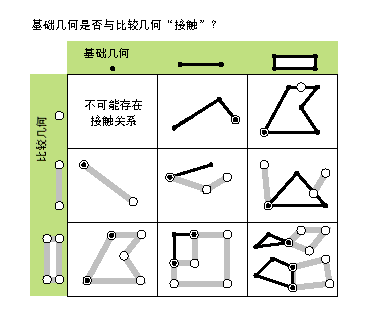union (other) 构造一个几何体，该几何体是输入几何的并集。要合并的两个几何必须为同一 shape 类型。within (second_geometry, {relation}) 指明基础几何是否位于比较几何之内。within 与运算符 contains 相反。本图仅显示 True 关系。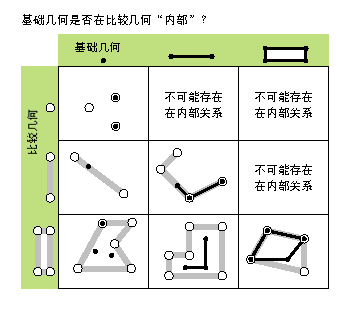如果基础几何是这些几何的交集且其内部交集不为空，则基础几何位于比较几何之内。within 是 Clementini 运算符，空的基础几何除外。

## 方法

`angleAndDistanceTo (other, {method})`
 参数 说明 数据类型 other The second geometry. If the geometry is a polygon, the distance is measured to the centroid of the polygon. PointGeometry method The method used to measure distance. GEODESIC—The shortest line between any two points on the earth's surface on a spheroid (ellipsoid). One use for a geodesic line is to determine the shortest distance between two cities for an airplane's flight path. This is also known as a great circle line if based on a sphere rather than an ellipsoid.GREAT_ELLIPTIC—The line on a spheroid (ellipsoid) defined by the intersection at the surface by a plane that passes through the center of the spheroid and the start and endpoints of a segment. This is also known as a great circle when a sphere is used.LOXODROME—The line follows a single compass bearing, or azimuth. Great circle routes are often broken into a series of loxodromes, which simplifies navigation. This is also known as a rhumb line.PLANAR—Planar measurements use 2D Cartesian mathematics to calculate lengths and areas. This option is only available when measuring in a projected coordinate system and the 2D plane of that coordinate system will be used as the basis for the measurements. Planar measurements reflect the projection of geographic data onto a 2D surface, and do not account for the curvature of the earth.PRESERVE_SHAPE—This type calculates the area or length of the geometry on the surface of the earth ellipsoid, for geometry defined in a projected or geographic coordinate system. This option preserves the shape of the geometry in its coordinate system.(默认值为 GEODESIC) String

 数据类型 说明 tuple 将一组角（以度为单位）和距离（以米为单位）返回到另一个点。
`boundary ()`

 数据类型 说明 Object 面的边界为折线。折线的边界为多点，与线的端点相对应。点或多点的边界为空点或多点。
`buffer (distance)`
 参数 说明 数据类型 distance 缓冲距离。缓冲几何时，缓冲距离采用相同的单位。仅可以为面几何指定负距离。 Double

 数据类型 说明 Polygon 缓冲的面几何。
`clip (envelope)`
 参数 说明 数据类型 envelope An Extent object used to define the clip extent. Extent

 数据类型 说明 Object 将输出几何体按指定范围进行裁剪。
`contains (second_geometry, {relation})`
 参数 说明 数据类型 second_geometry 第二个几何。 Object relation 空间关系类型。BOUNDARY— 关系对内部或边界没有任何限制。CLEMENTINI— 几何内部必须相交。指定 CLEMENTINI 等于指定 None。这是默认设置。PROPER— 几何边界不得相交。(默认值为 None) String

 数据类型 说明 Boolean 返回的布尔值 True 表示该几何包含第二个几何。
`convexHull ()`

 数据类型 说明 Object 生成的几何。单个点的凸包为该点本身。
`crosses (second_geometry)`
 参数 说明 数据类型 second_geometry 第二个几何。 Object

 数据类型 说明 Boolean 返回布尔值为 True 指明两个几何相交于较小形状类型的几何。
`cut (cutter)`
 参数 说明 数据类型 cutter 剪切折线几何。 PolyLine

 数据类型 说明 Geometry 包含两个几何的列表。
`densify (type, distance, {deviation})`
 参数 说明 数据类型 type 增密、DISTANCE、ANGLE 或 GEODESIC 的类型。 DISTANCE—创建输入分段线性近似的新要素。 ANGLE—创建输入分段线性近似的新要素。折点在以下点处引入：点处切线间的角度为提供的 angle。 GEODESIC—增密和修整输入折点间的线段，从而使输出线段可以随着连接输入折点的最短地面路径移动。 String distance 折点间的最大距离。折点间的实际距离通常小于最大距离，原因是新折点会沿着原始线段均匀分布。 如果使用 DISTANCE 的 type 或 ANGLE，则距离将以几何空间参考的单位进行测量。如果使用 GEODESIC 的 type，则用米作单位测量距离。 Double deviation 增密使用直线逼近曲线。可以使用 deviation 控制近似精度。偏差是新线段与原始曲线间的最大距离。其值越小，就需要越多的线段来逼近曲线。 如果使用 DISTANCE 的 type，则用几何空间参考单位测量偏差。如果使用 ANGLE 的 type，则以弧度为单位来测量偏差。如果使用 GEODESIC 的 type，则不使用偏差。 Double

 数据类型 说明 Geometry 增密几何。
`difference (other)`
 参数 说明 数据类型 other 第二个几何。 Object

 数据类型 说明 Object 生成的几何。
`disjoint (second_geometry)`
 参数 说明 数据类型 second_geometry 第二个几何。 Object

 数据类型 说明 Boolean 返回布尔值 True 表示两个几何未共用任何点。
`distanceTo (other)`
 参数 说明 数据类型 other 第二个几何。 Object

 数据类型 说明 Double 两个几何之间的距离。
`equals (second_geometry)`
 参数 说明 数据类型 second_geometry 第二个几何。 Object

 数据类型 说明 Boolean 返回布尔值为 True 表示两个几何的 shape 类型相同并在平面中定义了相同点集。
`generalize (max_offset)`
 参数 说明 数据类型 max_offset 最大偏移容差。 Double

 数据类型 说明 Geometry 概化的几何。
`getArea ({type}, {units})`
 参数 说明 数据类型 type The method used to measure area. GEODESIC—The shortest line between any two points on the earth's surface on a spheroid (ellipsoid). One use for a geodesic line is to determine the shortest distance between two cities for an airplane's flight path. This is also known as a great circle line if based on a sphere rather than an ellipsoid.GREAT_ELLIPTIC—The line on a spheroid (ellipsoid) defined by the intersection at the surface by a plane that passes through the center of the spheroid and the start and endpoints of a segment. This is also known as a great circle when a sphere is used.LOXODROME—The line follows a single compass bearing, or azimuth. Great circle routes are often broken into a series of loxodromes, which simplifies navigation. This is also known as a rhumb line.PLANAR—Planar measurements use 2D Cartesian mathematics to calculate lengths and areas. This option is only available when measuring in a projected coordinate system and the 2D plane of that coordinate system will be used as the basis for the measurements. Planar measurements reflect the projection of geographic data onto a 2D surface, and do not account for the curvature of the earth.PRESERVE_SHAPE—This type calculates the area or length of the geometry on the surface of the earth ellipsoid, for geometry defined in a projected or geographic coordinate system. This option preserves the shape of the geometry in its coordinate system.(默认值为 GEODESIC) String units The units in which the area will be calculated.ACRES—AcresARES—AresHECTARES—HectaresSQUARECENTIMETERS—Square centimetersSQUAREDECIMETERS—Square decimetersSQUAREFEET—Square feetSQUAREINCHES—Square inchesSQUAREKILOMETERS—Square kilometersSQUAREMETERS—Square metersSQUAREMILES—Square milesSQUAREMILLIMETERS—Square millimetersSQUAREYARDS—Square yards String

 数据类型 说明 Double 要素的面积。默认情况下，在投影坐标系中，将采用坐标系的单位返回面积，在地理坐标系中，将以平方米为单位返回面积。
`getLength ({measurement_type}, {units})`
 参数 说明 数据类型 measurement_type The method used to measure length. GEODESIC—The shortest line between any two points on the earth's surface on a spheroid (ellipsoid). One use for a geodesic line is to determine the shortest distance between two cities for an airplane's flight path. This is also known as a great circle line if based on a sphere rather than an ellipsoid.GREAT_ELLIPTIC—The line on a spheroid (ellipsoid) defined by the intersection at the surface by a plane that passes through the center of the spheroid and the start and endpoints of a segment. This is also known as a great circle when a sphere is used.LOXODROME—The line follows a single compass bearing, or azimuth. Great circle routes are often broken into a series of loxodromes, which simplifies navigation. This is also known as a rhumb line.PLANAR—Planar measurements use 2D Cartesian mathematics to calculate lengths and areas. This option is only available when measuring in a projected coordinate system and the 2D plane of that coordinate system will be used as the basis for the measurements. Planar measurements reflect the projection of geographic data onto a 2D surface, and do not account for the curvature of the earth.PRESERVE_SHAPE—This type calculates the area or length of the geometry on the surface of the earth ellipsoid, for geometry defined in a projected or geographic coordinate system. This option preserves the shape of the geometry in its coordinate system.(默认值为 GEODESIC) String units The units in which the length will be calculated.CENTIMETERS—CentimetersDECIMETERS—DecimetersFEET—FeetINCHES—InchesKILOMETERS—KilometersMETERS—MetersMILES—MilesMILLIMETERS—MillimetersNAUTICALMILES—Nautical MilesYARDS—Yards String

 数据类型 说明 Double 要素的长度。默认情况下，在投影坐标系中，将采用坐标系的单位返回长度，在地理坐标系中，将以米为单位返回长度。
`getPart ({index})`
 参数 说明 数据类型 index The index position of the geometry. Integer

 数据类型 说明 Array 生成的 Array 对象。
`intersect (other, dimension)`
 参数 说明 数据类型 other 第二个几何。 Object dimension 生成几何的拓扑维度（形状类型）。1—零维几何（点或多点）。2—一维几何（折线）。4—二维几何（面）。 Integer

 数据类型 说明 Object 作为两个输入几何体的交集的新几何体（点、多点、折线或面）。
`measureOnLine (in_point, {use_percentage})`
 参数 说明 数据类型 in_point 用于从折线的起点开始测量的点（PointGeometry 或 Point）。 PointGeometry use_percentage 如果 False，则测量值将作为距离返回；如果True，则测量值将作为百分比返回。(默认值为 False) Boolean

 数据类型 说明 Double 一个距离或百分比。
`overlaps (second_geometry)`
 参数 说明 数据类型 second_geometry 第二个几何。 Object

 数据类型 说明 Boolean 返回布尔值为 True 表示两个几何的交集具有与其中一个输入几何相同的尺寸。
`pointFromAngleAndDistance (angle, distance, {method})`
 参数 说明 数据类型 angle 到返回点的角度（以度为单位）。 Double distance 到返回点的距离（采用几何空间参考的单位）。 Double method PLANAR 测量值反映出的是地理数据在 2D 表面上的投影（也就是说，这些测量值不考虑地球的曲率）。可根据需要选择 GEODESIC、GREAT_ELLIPTIC、LOXODROME 或 PRESERVE_SHAPE 测量类型作为替代类型。GEODESIC—椭球体（椭圆体）地球表面上任意两点间长度最短的线。要确定两城市间最短的飞机飞行路径，便会用到大地测量线。如果基于一个球体而非一个椭圆体，则这种线又称为大圆线。GREAT_ELLIPTIC—椭球体（椭圆体）上的线，定义为通过椭球体中心以及某线段的起点和终点的平面与椭球体相交产生的线。当使用球体时，此形状又称为大圆。LOXODROME—斜航线并非两点之间的最短距离，而是定义固定方位或方向的线。大圆路径经常被分解成一系列斜航线，以简化导航过程。它又称为等角航线 (rhumb line)。PLANAR—平面测量使用 2D 笛卡尔算法计算长度与面积。此选项仅适用于在投影坐标系中执行测量，并且该坐标系的 2D 平面将作为测量的基础。PRESERVE_SHAPE—该类型可计算地球椭圆体表面几何的面积或长度，其中几何已在投影坐标系或地理坐标系中定义。此选项保留了几何在坐标系中的形状。(默认值为 GEODESIC) String

 数据类型 说明 PointGeometry 按给定的角度（以度为单位）和距离（以米为单位）返回点。
`positionAlongLine (value, {use_percentage})`
 参数 说明 数据类型 value 沿线的距离。如果距离小于零，则将返回线的起点；如果距离大于线的长度，则将返回线的终点。 Double use_percentage 可将距离指定为测量的固定单位或线的长度比率。如果为真，则 value 将被用作百分比，如果为假，则 value 将被用作距离。对于百分比，该值应表示为 0.0 (0％) 到 1.0 (100％) 的双精度。(默认值为 False) Boolean

 数据类型 说明 PointGeometry 线上距线起点指定距离的点。
`projectAs (spatial_reference, {transformation_name})`
 参数 说明 数据类型 spatial_reference The new spatial reference. This can be a SpatialReference object or the coordinate system name. SpatialReference transformation_name The geotransformation name. String

 数据类型 说明 Object 经投影的几何。
`queryPointAndDistance (in_point, {as_percentage})`
 参数 说明 数据类型 in_point 输入点（PointGeometry 或 Point）。 PointGeometry as_percentage 如果 False，会将测量值作为距离返回；如果 True，将以百分比返回测量值。(默认值为 False) Boolean

 数据类型 说明 tuple 返回包含以下信息的一个元组：PointGeometry，折线上距 in_point 最近的点。线的起点和返回点之间的距离。线和 in_point 间的最小距离。指示 in_point 是否在线右侧的布尔值。线的方向决定左右两侧。
`segmentAlongLine (start_measure, end_measure, {use_percentage})`
 参数 说明 数据类型 start_measure 从线起点起的起始距离。 Double end_measure 从线起点起的结束距离。 Double use_percentage 可将起始和结束测量值指定为固定单位或比率。如果为真，则 start_measure 和 end_measure 将被用作百分比，如果为假，则 start_measure 和 end_measure 将被用作距离。对于百分比，该测量值应表示为 0.0 (0％) 到 1.0 (100％) 的双精度。(默认值为 False) Boolean

 数据类型 说明 PolyLine 两点之间的线段。
`snapToLine (in_point)`
 参数 说明 数据类型 in_point 要捕捉到该线的点（PointGeometry 或 Point）。 PointGeometry

 数据类型 说明 PointGeometry 捕捉到的点。
`symmetricDifference (other)`
 参数 说明 数据类型 other 第二个几何。 Object

 数据类型 说明 Object 生成的几何。
`touches (second_geometry)`
 参数 说明 数据类型 second_geometry 第二个几何。 Object

 数据类型 说明 Boolean 返回布尔值为 True，表示几何的边界相交。
`union (other)`
 参数 说明 数据类型 other 第二个几何。 Object

 数据类型 说明 Object 生成的几何。
`within (second_geometry, {relation})`
 参数 说明 数据类型 second_geometry 第二个几何。 Object relation 空间关系类型。BOUNDARY— 关系对内部或边界没有任何限制。CLEMENTINI— 几何内部必须相交。指定 CLEMENTINI 等于指定 None。这是默认设置。PROPER— 几何边界不得相交。(默认值为 None) String

 数据类型 说明 Boolean 返回布尔值 True 表示该几何被包含在另一个几何之内。

## 代码示例

``````import arcpy

# Run the Copy Features tool, setting the output to the geometry object.
# geometries is returned as a list of geometry objects.
geometries = arcpy.CopyFeatures_management("c:/data/streets.shp",
arcpy.Geometry())

# Walk through each geometry, totaling the length
length = 0
for geometry in geometries:
length += geometry.length

print("Total length: {0}".format(length))``````